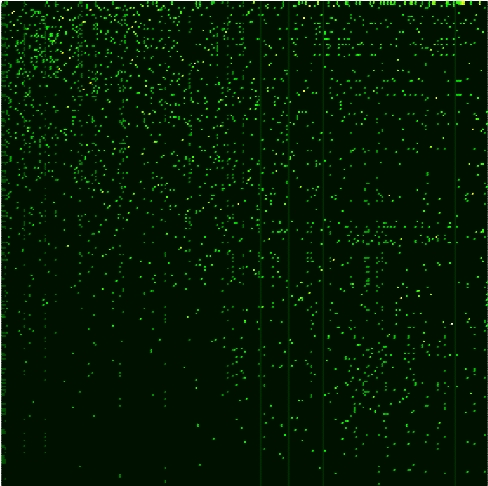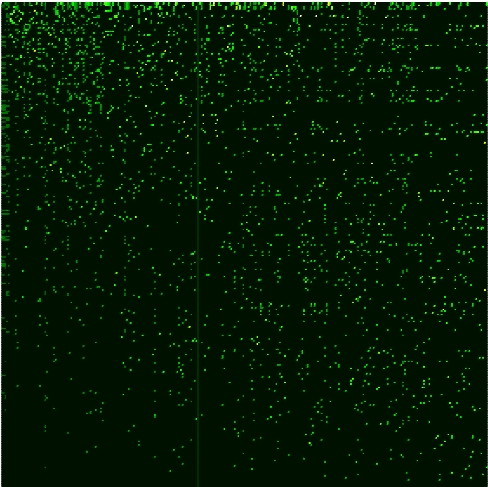Google matrix analysis of C.elegans neural network
by V.Kandiah and D.L.Shepelyansky
arXiv:1311.2013` `Article download (here)Published article Phys. Lett. A v.378, p.1932 (2014) (here)Neuron indexes and names (here), neuron ranks (here)Neurons in order of PageRank (here), CheiRank (here), 2DRank (here)Links: synaptic connections (here), gap connections (here), sum of links (here)Google matrix elements of G (here)Google matrix elements of G* (here)Tables of top 20 elements of eigenstates with 50 largest modulus of eigenvalues (here)Table of PageRank (PR), CheiRank (CR), equal oportunity PageRank (EQOPR), equal opertunity CheiRank (EQOCR) with 50 largest modulus of eigenvalues, colors are as in right panel of Fig.5 (here)This paper and web page should be cited if these data are used This webpage is created at November 8, 2013 and is maintained by V.Kandiah and D.L.Shepelyansky